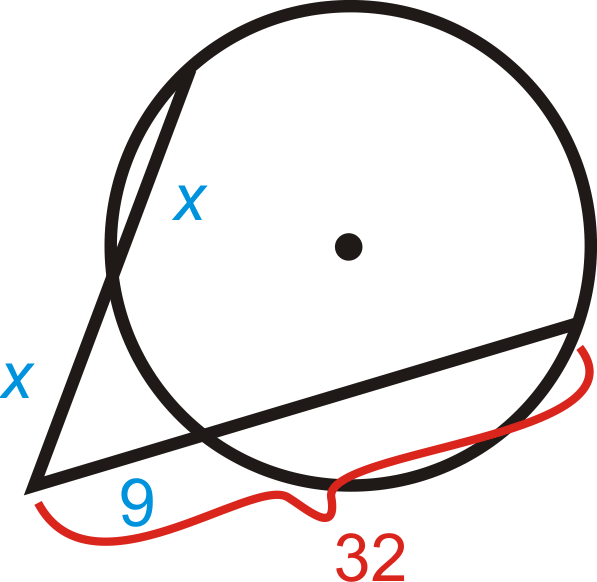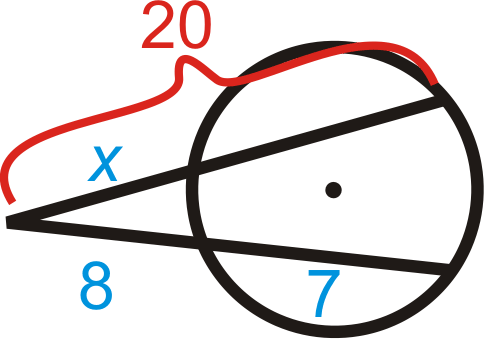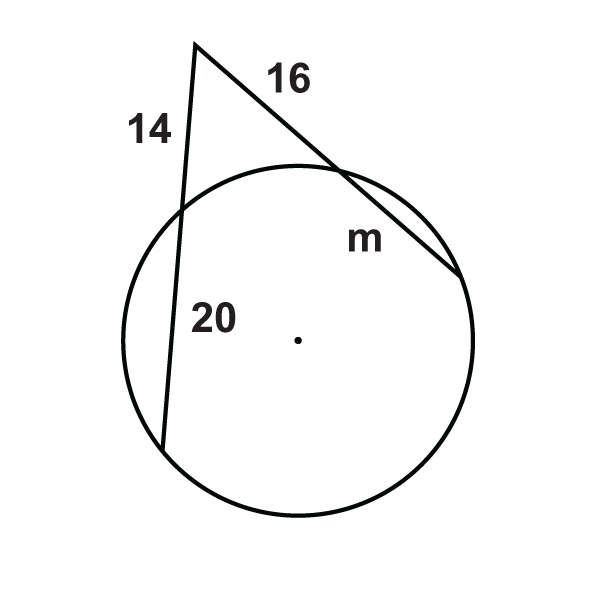# 6.19: Intersecting Secants Theorem

•• Contributed by CK12
• CK12

Prove and use theorems involving lines that intersect a circle at two points.

## Segments from Secants

When two secants intersect outside a circle, the circle divides the secants into segments that are proportional with each other.

Two Secants Segments Theorem: If two secants are drawn from a common point outside a circle and the segments are labeled as below, then $$a(a+b)=c(c+d)$$.Figure $$\PageIndex{1}$$

What if you were given a circle with two secants that intersect outside the circle? How could you use the length of some of the segments formed by their intersection to determine the lengths of the unknown segments?

Example $$\PageIndex{1}$$

Find $$x$$. Simplify any radicals.Figure $$\PageIndex{2}$$

Solution

Use the Two Secants Segments Theorem.

\begin{aligned} 8(8+x)&=6(6+18) \\ 64+8x&=144 \\ 8x&=80 \\ x&=10\end{aligned}

Example $$\PageIndex{2}$$

Find $$x$$. Simplify any radicals.Figure $$\PageIndex{3}$$

Solution

Use the Two Secants Segments Theorem.

\begin{aligned} 15(15+27)&=x\cdot45 \\ 630&=45x \\ x&=14 \end{aligned}

Example $$\PageIndex{3}$$

Find the value of $$x$$.Figure $$\PageIndex{4}$$

Solution

Use the Two Secants Segments Theorem.

\begin{aligned}18\cdot(18+x)&=16\cdot(16+24) \\ 324+18x&=256+384 \\ 18x&=316 \\ x&=17\dfrac{5}{9}\end{aligned}

Example $$\PageIndex{4}$$

Find the value of $$x$$.Figure $$\PageIndex{5}$$

Solution

Use the Two Secants Segments Theorem.

\begin{aligned}x\cdot(x+x)&=9\cdot 32 \\ 2x^2&=288 \\ x^2&=144 \\ x&=12,\: x\neq −12 (\text{length is not negative})\end{aligned}

Example $$\PageIndex{5}$$

True or False: Two secants will always intersect outside of a circle.

Solution

False. If the two secants are parallel, they will never intersect. It's also possible for two secants to intersect inside a circle.

## Review

Fill in the blanks for each problem below. Then, solve for the missing segment.

1.Figure $$\PageIndex{6}$$

$$3(\text{______}+\text{______})=2(2+7)$$

1.Figure $$\PageIndex{7}$$

$$x\cdot\text{______}=8(\text{______}+\text{______})$$

Find x in each diagram below. Simplify any radicals.

1.Figure $$\PageIndex{8}$$
2.Figure $$\PageIndex{9}$$
3.Figure $$\PageIndex{10}$$
1. Fill in the blanks of the proof of the Two Secants Segments Theorem.Figure $$\PageIndex{11}$$

Given: Secants $$\overline{PR}$$ and $$\overline{RT}$$

Prove: $$a(a+b)=c(c+d)$$

Statement Reason
1. Secants $$\overline{PR}$$ and $$\overline{RT}$$ with segments $$a$$, $$b$$, $$c$$, and $$d$$. 1. Given
2. $$\angle R\cong \angle R$$ 2. Reflexive PoC
3. $$\angle QPS\cong \angle STQ$$ 3. Congruent Inscribed Angles Theorem
4. $$\Delta RPS\sim \Delta RTQ$$ 4. AA Similarity Postulate
5. $$ac+d=ca+b$$ 5. Corresponding parts of similar triangles are proportional
6. $$a(a+b)=c(c+d)$$ 6. Cross multiplication

Solve for the unknown variable.

1.Figure $$\PageIndex{12}$$
2.Figure $$\PageIndex{13}$$
3.Figure $$\PageIndex{14}$$
4.Figure $$\PageIndex{15}$$
5.Figure $$\PageIndex{16}$$
6.Figure $$\PageIndex{17}$$
7.Figure $$\PageIndex{18}$$
8.Figure $$\PageIndex{19}$$
9.Figure $$\PageIndex{12}$$

## Vocabulary

Term Definition
central angle An angle formed by two radii and whose vertex is at the center of the circle.
chord A line segment whose endpoints are on a circle.
circle The set of all points that are the same distance away from a specific point, called the center.
diameter A chord that passes through the center of the circle. The length of a diameter is two times the length of a radius.
Inscribed Angle An inscribed angle is an angle with its vertex on the circle. The measure of an inscribed angle is half the measure of its intercepted arc.
intercepted arc The arc that is inside an inscribed angle and whose endpoints are on the angle.
point of tangency The point where the tangent line touches the circle.
radius The distance from the center to the outer rim of a circle.
AA Similarity Postulate If two angles in one triangle are congruent to two angles in another triangle, then the two triangles are similar.
Congruent Congruent figures are identical in size, shape and measure.
Reflexive Property of Congruence $$\overline{AB}\cong \overline{AB}$$ or $$\angle B\cong \angle B$$
Secant The secant of an angle in a right triangle is the value found by dividing length of the hypotenuse by the length of the side adjacent the given angle. The secant ratio is the reciprocal of the cosine ratio.
secant line A secant line is a line that joins two points on a curve.
Tangent line A tangent line is a line that "just touches" a curve at a single point and no others.
Two Secants Segments Theorem Two secants segments theorem states that if you have a point outside a circle and draw two secant lines from it, there is a relationship between the line segments formed.